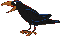### FITUG e.V.

##### Förderverein Informationstechnik und Gesellschaft# New NSA Patent: Method of passing a cryptographic key that allows third party access to the key

Method of passing a cryptographic key that allows third party access to the key

Abstract

A method of passing a cryptographic key that allows recovery of the key by a third party by generating a first random number by a first user; generating "key.sub.1 " by the first user; generating a second random number "k.sub.2a " by the first user; computing "y.sub.1 " by the first user; computing "y.sub.2 " by the first user; computing "r.sub.1 " by the first user; computing "z" by the first user; computing "s" by the first user; computing "G" by the first user; passing (G,z,r.sub.1,s) from the first user to the second user; receiving "Y" by the second user; computing "T" by the second user; computing "y.sub.1 " by the second user; computing "k.sub.1a " by the second user; computing "key.sub.1 " by the second user; intercepting, by a third party, (G,z,r.sub.1,s) transmitted from the first user to the second user; presenting "G" and "z," by the third party, to a key- escrow agent; computing "y.sub.2 " by the key-escrow agent; computing "key.sub.2 " by the key-escrow agent, where key.sub.2 =key.sub.1 ; returning "key" from the key-escrow agent to the third party if the third party is authorized to receive "key.sub.2 "; and using "key.sub.2 " by the authorized third party, to decrypt an encrypted message sent between the first user and the second user which was encrypted using "key.sub.1."

Inventors: Petro; John (Columbia, MD) Assignee: The United States of America as represented by the National Security Agency (Washington, DC) Appl. No.: 722385 Filed: October 11, 1996

Current U.S. Class: 380/21; 380/23 Intern'l Class: H04K 001/00 Field of Search: 380/21,23,28-30,285,286

[...]

What is claimed is:

1. A method of passing a cryptographic key that allows recover of the key by a third party, comprising the steps of:

a) generating a first random number "k.sub.1a " by a first user, where "k.sub.1a " is a non-zero member of a group "Z.sub.q " and where "q" is a prime number;

b) generating "key.sub.1 =m(k.sub.1a)" by the first user, where "m" is a hashing function;

c) generating a second random number "k.sub.2a " by the first user, where "k.sub.2a " is a non-zero member of the group "Z.sub.q ";

d) computing "y.sub.1 =m(h.sub.p2 (k.sub.2a))" by the first user, where "h.sub.p2 " is a public function of a public-key encryption function of a second user;

e) computing "y.sub.2 =m(H.sub.p (k.sub.1a))" by the first user, where "H.sub.p " is a public function of a public-key encryption function of a key escrow agent;

f) computing "r.sub.1 =f(y.sub.1,k.sub.1a)" by the first user, where "f" is a secure encryption function;

g) computing "z=f(y.sub.2, key.sub.1)" by the first user;

h) computing a signature, by the first user, by computing "A=(1/k.sub.2a)(x.sub.a B+k.sub.1a C)mod q,"where "z", "r.sub.1 ", and "s" are substituted for "A", "B", and "C," where the equation for computing a signature is solved for "s," and where "x.sub.a " is a long-term secret of the first user;

i) computing "G=g k.sub.1a mod p" by the first user;

j) passing (G,z,r.sub.1,s) from the first user to the second user;

k) receiving "Y=g x.sub.a mod p" by the second user, where "g" is a base element, and where "p" is a prime integer;

l) verifying the computed signature, by the second user, by computing "T=(Y.sup.B *G.sup.C).sup.((1/A) mod q) mod p," where "z", "r.sub.1 ", and "s" are substituted for "A", "B", and "C";

m) computing "y.sub.1 =m(h.sub.s2 (T))" by the second user, where "h.sub.s2 " is a secret function of the public-key encryption function of the second user;

n) computing "k.sub.1a =(f.sup.-1)(y.sub.1,r.sub.1)" by the second user;

o) computing "key.sub.1 =m(k.sub.1a)" by the second user;

p) intercepting, by a third party, (G,z,r.sub.1,s) transmitted from the first user to the second user;

q) presenting "G" and "z," by the third party, to a key-escrow agent;

r) computing "y.sub.2 =m(H.sub.s (G))" by the key-escrow agent, where "H.sub.s " is a secret function of the public-key encryption function of the key-escrow agent;

s) computing "key.sub.2 =(f.sup.-1)(y.sub.2, z)" by the key-escrow agent, where key.sub.2 =key.sub.1 ;

t) returning "key.sub.2 " from the key-escrow agent to the third party if the third party is authorized to receive "key.sub.2 "; and

u) using "key.sub.2," by the authorized third party, to decrypt an encrypted message sent between the first user and the second user which was encrypted using "key.sub.1 ".

[...]